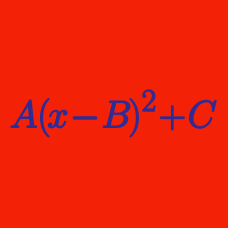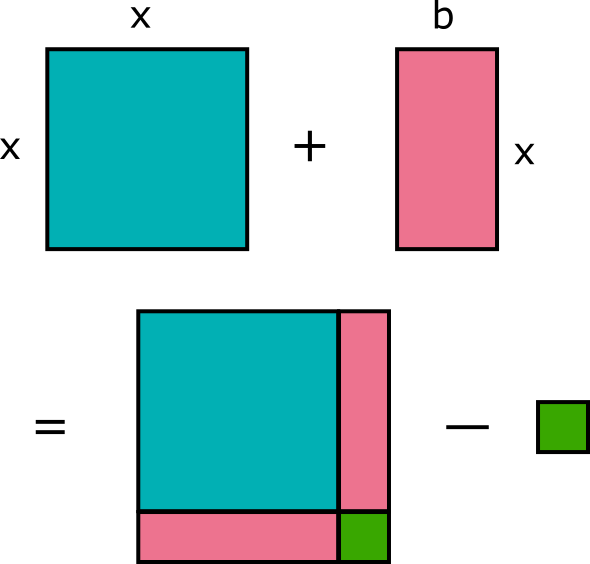Algebra

# Completing the Square Warmup

$x^2 + 8x + 16 = (x + B)^2$

What is the value of $B?$

Which of the following is equivalent to $x^2 + 6x + 10?$Given that the green portion is a square, what is its area?

What are the solutions of

$x^2 + 8x + 7 = 0?$

$(x - 3)^2 = 25$

What are the solutions to this equation?

Hint:

$\sqrt{(x - 3)^2} = \pm \sqrt{25}$

×